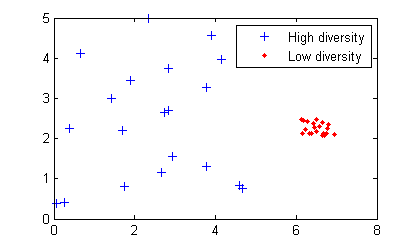## Genetic Algorithm Terminology

### Fitness Functions

The fitness function is the function you want to optimize. For standard optimization algorithms, this is known as the objective function. The toolbox software tries to find the minimum of the fitness function.

Write the fitness function as a file or anonymous function, and pass it as a function handle input argument to the main genetic algorithm function.

### Individuals

An individual is any point to which you can apply the fitness function. The value of the fitness function for an individual is its score. For example, if the fitness function is

`$f\left({x}_{1},{x}_{2},{x}_{3}\right)={\left(2{x}_{1}+1\right)}^{2}+{\left(3{x}_{2}+4\right)}^{2}+{\left({x}_{3}-2\right)}^{2},$`

the vector (2, -3, 1), whose length is the number of variables in the problem, is an individual. The score of the individual (2, –3, 1) is f(2, –3, 1) = 51.

An individual is sometimes referred to as a genome and the vector entries of an individual as genes.

### Populations and Generations

A population is an array of individuals. For example, if the size of the population is 100 and the number of variables in the fitness function is 3, you represent the population by a 100-by-3 matrix. The same individual can appear more than once in the population. For example, the individual (2, -3, 1) can appear in more than one row of the array.

At each iteration, the genetic algorithm performs a series of computations on the current population to produce a new population. Each successive population is called a new generation.

### Diversity

Diversity refers to the average distance between individuals in a population. A population has high diversity if the average distance is large; otherwise it has low diversity. In the following figure, the population on the left has high diversity, while the population on the right has low diversity.Diversity is essential to the genetic algorithm because it enables the algorithm to search a larger region of the space.

### Fitness Values and Best Fitness Values

The fitness value of an individual is the value of the fitness function for that individual. Because the toolbox software finds the minimum of the fitness function, the best fitness value for a population is the smallest fitness value for any individual in the population.

### Parents and Children

To create the next generation, the genetic algorithm selects certain individuals in the current population, called parents, and uses them to create individuals in the next generation, called children. Typically, the algorithm is more likely to select parents that have better fitness values.

Watch now